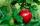# Expression of a variable from the formula - math word problems

1. R TrapeziumRectangular trapezium has bases 12 and 5 and area 84 cm2. What is its perimeter?
2. Arc and segmentCalculate the length of circular arc l, area of the circular arc S1 and area of circular segment S2. Radius of the circle is 33 and corresponding angle is ?.
3. Prism XThe prism with the edges of the lengths x cm, 2x cm and 3x cm has volume 20250 cm3. What is the area of surface of the prism?
4. ISO triangleCalculate the area of an isosceles triangle KLM if the length of its sides are in the ratio k:l:m = 4:4:3 and has perimeter 377 mm.
5. GardenFather dig up the garden in 9 hours. Son in 13 hours. How many hours take dig up the garden together?
6. Rhombus 2Calculate the area of rhombus which has a height v=48 mm and shorter diagonal u = 60 mm long.
7. CircleHow big is area of circle if its circumference is 80.6 cm?
8. Right isoscelesCalculate area of the isosceles right triangle which perimeter is 41 cm.
9. Square diagonalCalculate the length of diagonal of the square with side a = 23 cm.
10. TrianglesEquilateral triangle with side 40 cm has the same perimeter as an isosceles triangle with arm of 45 cm. Calculate the base x of an isosceles triangle.The rectangular crossroads comes passenger car and an ambulance, the ambulance left. Passenger car is at 36 km/h and ambulance 47 km/h. Calculate such a relative speed of the ambulance moves to the car.
12. GardenTrapezoid garden has parallel sides 19 m and 24 m. Its area is 193.5 square meters. What is the width of the garden?
13. Cylinder - hCylinder volume is 215 cm3. Base radius is 2 cm. Calculate the height of the cylinder.
14. Truncated coneCalculate the height of the rotating truncated cone with volume V = 1115 cm3 and a base radii r1 = 7.9 cm and r2 = 9.7 cm.
15. Right triangleCalculate the missing side b and interior angles, perimeter and area of ​​a right triangle if a=10 cm and hypotenuse c = 16 cm.
16. Perimeter and legsDetermine the perimeter of a right triangle if the length of one leg is 75% length of the second leg and its content area is 24 cm2.
17. Sphere A2VSurface of the sphere is 241 mm2. What is its volume?
18. Hollow sphereSteel hollow sphere floats on the water plunged into half its volume. Determine the outer radius of the sphere and wall thickness, if you know that the weight of the sphere is 0.5 kg and density of steel is 7850 kg/m3
19. Cone and the ratioRotational cone has a height 23 cm and the ratio of the base surface to lateral surface is 7: 9. Calculate a surface of the base and the lateral surface.
20. Spherical segmentSpherical segment with height h=7 has a volume V=198. Calculate the radius of the sphere of which is cut this segment.

Do you have an interesting mathematical word problem that you can't solve it? Enter it, and we can try to solve it.

To this e-mail address, we will reply solution; solved examples are also published here. Please enter the e-mail correctly and check whether you don't have a full mailbox.

Please do not submit problems from current active competitions such as Mathematical Olympiad, correspondence seminars etc...